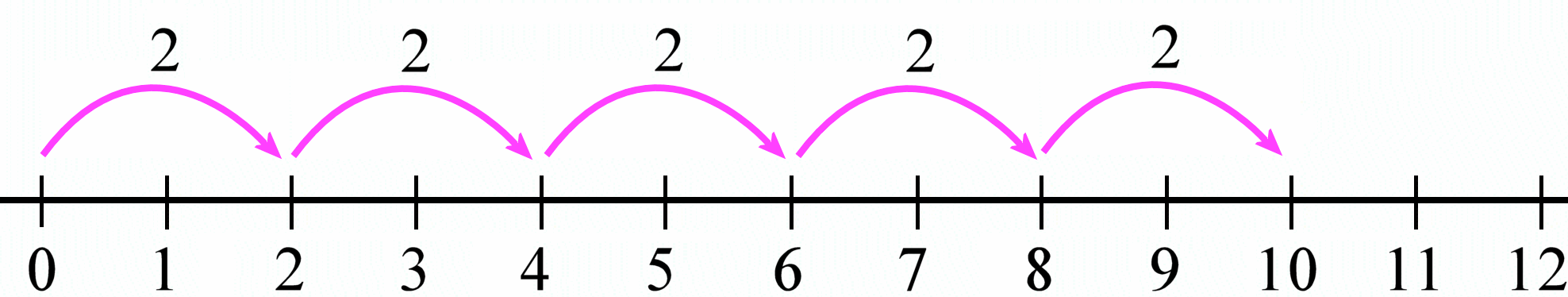×

Search anything:

# Range Function in Python

#### Python Software Engineering rangeRange is an inbuilt function in python that is generally used to create a sequence of numbers. It can accept upto three arguments to modify the output accordingly. We will look into its functionalities and any limitations that range has. Let's start from basics and build up our understanding of range.

## Simplest usage of range()

Fire up a python shell and type the following.

``````>>> for i in range(7):
...     print(i)
...
0
1
2
3
4
5
6
``````

When range is called with only one argument it generates a sequence from 0 upto the number (but not included) specified.

The goal of using `range(x)` in a for loop is that the loop will iterate exactly x times. Hence, we get numbers from 0 to x-1.

## I don't want to start from 0

No problem. That is as simple as passing two values to range() function. The first argument will tell from where to start iterating and the second argument will tell where to stop just like the previous example.

``````>>> for i in range(8, 12):
...     print(i) # or any other operation
...
8
9
10
11
``````

Can you guess how many times the loop will iterate in this case? Yeah, the code inside the for loop will execute four times in this particular example.

Using `range(x, y)` with a for loop will make it iterate "y-x" times. Hence, `i` will be assigned numbers from x to y-1.

We can also pass negative values to x and y. Just make sure that x is smaller than y because we are iterating from a smaller number to a big number.

``````# prints -6, -5, -4, -3, -2, -1, 0, 1
for i in range(-6, 2):
print(i)

# prints -7, -6, -5, -4
for i in range(-7, -3):
print(i)

# prints nothing
for i in range(-11, -15):
print(i)
``````

## Skipping StonesYou may be familiar with this kind of image from your math books. The same can be done with range function. We can tell it by how much to increment by passing in a third argument.

``````>>> for i in range(0, 21, 2):
...    print(i)
...
0
2
4
6
8
10
12
14
16
18
20
``````

Quick and easy way to generate multiplication table of any number! No need to cram them anymore. That's what programming is all about. Solving problems in elegent ways.
Keep that picture in the back of your mind if you are confused what numbers range will spit out. By now you must have realised that the default value passed in the third argument is 1.

In this case i.e., `range(x, y, z)` the for loop will iterate `(y-x)/z` times (ignoring the decimal part).

## Counting in reverse

We are now starting to see the power of range function. To count in reverse direction just pass a negative number as z in `range(x, y, z)`. But here x must be greater than y because we are generating a sequence from bigger number towards a smaller number.

``````>>> for i in range(20, 14, -1):
...     print(i)
...
10
19
18
17
16
15
``````

Now try generating table of two in reverse i.e., from 20 to 0 yourself.

## What's with the `i`?

You may have noticed that we are using `i` everywhere in our examples. Well `i` is just a convention which is a shorthand for iterator. We can use any other valid variable name instead of `i`. It is recommended that local variables names are short and little give context of it's purpose. Chances are that we are not going to use `i` outside the for loop hence a single letter name will suffice.

## One last trick with range()

If you pass the output of range() function in list() it will return a list containing all the numbers.

``````>>> num = list(range(6))
>>> print(num)
[0, 1, 2, 3, 4, 5]
>>> type(num)
<class 'list'>
``````

We can also store the value returned by range() and use that inside a for loop instead.

``````iter = range(8)

# notice that we are using num instead of i
# same as for num in range(8)
for num in iter:
print(num)
``````

One possible drawback of range() function is that we cannot use it for iterating over float values because floats are not precise and hard to work with.

Range will only work with integer values.

This can be overcomed by using other programming tricks.

``````# prints numbers from 0.1 to 1.0
for i in range(1, 11):
print(i/10)
``````

## The end

That covers up the range function. Remember that you can always comment in the forum for any doubts and discussion. We will be more than happy to help you.